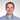# Queue Data Structure In JavaScript

July 15, 2018 - 5 min read

Queues are widely used in software for development when it’s necessary to share a resource among multiple consumers and for asynchronous data transfer (file IO, pipes, sockets). It also serves as an auxiliary data structure for algorithms and as a component of other data structures. Although, we can use JS arrays as queues, it’s a good brain-teaser to implement this data structure from scratch. This way we’ll understand the queue better and thus become better developers.

# Queue

The queue is the data structure that manages a collection of elements with two main operations:

• enqueue - which adds an element to the end of the queue;
• dequeue - which releases an element from the start of the queue.

We can think of the queue as of a line of people for checking in at the airport. The first coming to the line gets checked in the first. The last coming to the line gets checked in the last. So it’s the data structure representing FIFO (First In First Out) principle.

# Big-O Analysis

Here is the big-O analysis for the main operations:

• enqueue - O(1)
• dequeue - O(1)

But what is the big-O analysis for the operation of dequeueing the last element in the queue. Let’s recall our analogy with line of people and it becomes obvious that to process the last person in the line we have to process the whole line. So, the big-O complexity for this operation is O(N).

# Queue in JavaScript

As we already mentioned, we can use JS arrays as queues. Let’s illustrate this with an imaginary line of people.

``````const queue = []

// People are gathering in the line
queue.push("Adam (1st person in the line)")
queue.push("Lucy (2nd person in the line)")
queue.push("Tom (3rd person in the line)")
queue.push("Jerry (4th person in the line)")
queue.push("Mila (5th person in the line)")

// Check-in started
queue.shift() // -> "Adam (1st person in the line)" | Adam starts being registered, 4 people are waiting in the line
queue.shift() // -> "Lucy (2nd person in the line)" | Lucy starts being registered, 3 people are waiting in the line``````

# Queue Implementation

Let’s implement the queue by our own. We don’t want to emulate the implementation by creating a wrapper on top of the array. Instead, we are going to do it from scratch.

# Preliminary Considerations

Imagine that we’ve already created the queue. How would we use it then? Well, obviously, in the exact same manner as we did above:

``````const queue = new Queue()

// People are gathering in the line
queue.enqueue("Adam (1st person in the line)")
queue.enqueue("Lucy (2nd person in the line)")
queue.enqueue("Tom (3rd person in the line)")
queue.enqueue("Jerry (4th person in the line)")
queue.enqueue("Mila (5th person in the line)")

// Check-in started
queue.dequeue() // -> "Adam (1st person in the line)" | Adam starts being registered, 4 people are waiting in the line
queue.dequeue() // -> "Lucy (2nd person in the line)" | Lucy starts being registered, 3 people are waiting in the line``````

OK. Now we have a clear vision of what to implement. The next step is to figure out the internal storage mechanism. As with the stack, we’ll take a hash table as a storage.

We also have to be able to track the sequence of objects in the storage to correctly enqueue and dequeue objects out of it. When we implemented a stack, for this purpose we used one pointer - for the end of the stack. Now we’ll need two - one for the start of the queue and the other for its end.

# The solution

Here is a possible solution:

``````function Queue() {
const storage = {}
let start = 0
let end = 0

function enqueue(value) {
storage[end] = value
end++
}

function dequeue() {
if (getLength() === 0) {
return null
}
const v = storage[start]
delete storage[start]
start++
return v
}

function getValues() {
const result = []
for (let i = start; i < end; i++) {
result.push(storage[i])
}
return result
}

function getLength() {
return end - start
}

return { enqueue, dequeue, getValues, getLength }
}``````

Please notice that we added getValues() method returning a representation of the queue as an array from the first element in the queue to the last.

# Unit Tests

We like writing stable code, right? To avoid surprises we’re going to cover our queue with unit tests (Jest is used).

``````const { Queue } = require("./queue")
let values, q

beforeEach(() => {
values = [
"Adam (1st person in the line)",
"Lucy (2nd person in the line)",
"Tom (3rd person in the line)",
"Jerry (4th person in the line)",
"Mila (5th person in the line)"
]

q = new Queue()
values.forEach(v => q.enqueue(v))
})

test(`queues's 'enqueue' method works`, () => {
const qValues = q.getValues()

values.forEach((v, index) => {
expect(qValues[index]).toBe(values[index])
})

expect(q.getLength()).toBe(values.length)
})

test(`queues's 'dequeue' method works`, () => {
for (let i = 0; i < values.length; i++) {
expect(values[i]).toBe(q.dequeue())
}

expect(q.dequeue()).toBeNull()
expect(q.getValues().length).toBe(0)
})``````

# Conclusion

Queue is a fundamental data structure in software engineering. And now we can implement it from scratch in plain JS. Isn’t it great?

Happy coding!Written by Egor Panok, full stack JavaScript Developer who loves building useful things. Follow him on Twitter

© 2020, Egor Panok, Full Stack JavaScript Developer